Previous: 6.2.5 A Caveat on Up: 6.2 Quantum Mechanics in Next: 6.2.7 Quantum Propagators and

## 6.2.6 A Feasible Phase Space Distribution

There are numerical issues with the Wigner transform so that we would like to avoid this task. Instead we will now define a distribution which in one dimension classically approximates the phase space distribution. We want our distribution to reproduce exactly the marginal expectation values for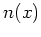,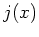and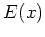. Other local expectation values are not necessarily reproduced correctly.

Carrier, current, and energy marginal densities can be directly calculated from the Schrödinger function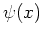respectively the hydrodynamical quantities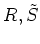with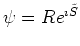. Instead of the densities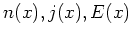we prefer to work with the marginal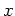-distributions for the in the case of constant mass equivalent set of local'' observables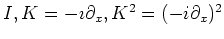, where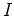denotes the identity operator.

Introducing the variable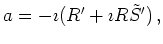(6.65)

we get the marginal expectation values (using the split of the energy operator as in Equation 6.63) for a pure state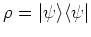: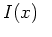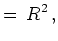(6.66)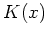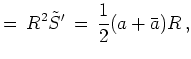(6.67)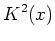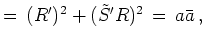(6.68)

To define a corresponding classical phase space distribution function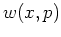we assume that for a wave function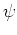the corresponding distribution w(x,p) for eachconsists of left (up) and right (down) going modes, i.e.: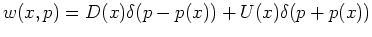(6.69)

with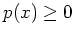.

This assumption makes sense in cases, in which the classical problem has a similar property. Such is the case for the scattering problems and the open Schrödinger equation which we consider in Section 7.1. In this case particles are injected with a fixed momentum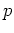from one electrode and condition 6.70 is fulfilled exactly. In the electrodes we have a superposition of transmitted and reflected modes.

From the ansatz 6.70 we can calculate the classical quantities: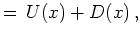(6.70)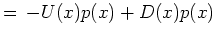(6.71)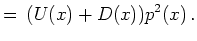(6.72)

Classically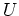and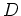are always positive quantities. Equating the marginal expectation values from the wave function and from the classical'' distribution function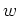we get the system of equations: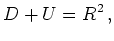(6.73)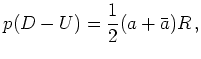(6.74)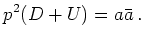(6.75)

From the last equation we get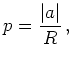(6.76)

which reduces the system of equations to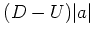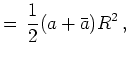(6.77)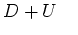(6.78)

with the solution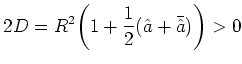(6.79)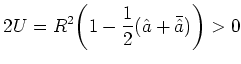(6.80)

with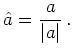(6.81)

The coefficientsandare positive values between 0 and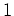as has to be the case for proper probabilities. To sum up: Our (1+1)-dimensional distribution function has the property that it is positive and reproduces the moments up to an order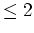correctly.